Simple Harmonic Motion NAT Level - 1

# Simple Harmonic Motion NAT Level - 1

Test Description

## 10 Questions MCQ Test Topic wise Tests for IIT JAM Physics | Simple Harmonic Motion NAT Level - 1

Simple Harmonic Motion NAT Level - 1 for IIT JAM 2023 is part of Topic wise Tests for IIT JAM Physics preparation. The Simple Harmonic Motion NAT Level - 1 questions and answers have been prepared according to the IIT JAM exam syllabus.The Simple Harmonic Motion NAT Level - 1 MCQs are made for IIT JAM 2023 Exam. Find important definitions, questions, notes, meanings, examples, exercises, MCQs and online tests for Simple Harmonic Motion NAT Level - 1 below.
Solutions of Simple Harmonic Motion NAT Level - 1 questions in English are available as part of our Topic wise Tests for IIT JAM Physics for IIT JAM & Simple Harmonic Motion NAT Level - 1 solutions in Hindi for Topic wise Tests for IIT JAM Physics course. Download more important topics, notes, lectures and mock test series for IIT JAM Exam by signing up for free. Attempt Simple Harmonic Motion NAT Level - 1 | 10 questions in 30 minutes | Mock test for IIT JAM preparation | Free important questions MCQ to study Topic wise Tests for IIT JAM Physics for IIT JAM Exam | Download free PDF with solutions
 1 Crore+ students have signed up on EduRev. Have you?
*Answer can only contain numeric values
Simple Harmonic Motion NAT Level - 1 - Question 1

### A spring has a certain mass suspended from it and its period for vertical oscillation is T1. The spring is now cut into two equal halves and the same mass is suspended from one of the half. The period of vertical oscillation is now T2. The ratio of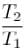is :

Detailed Solution for Simple Harmonic Motion NAT Level - 1 - Question 1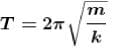When spring is cut in equal part, then force constant is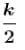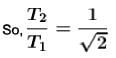*Answer can only contain numeric values
Simple Harmonic Motion NAT Level - 1 - Question 2

### For a simple harmonic vibrator of frequency n, the frequency of kinetic energy changing completely to potential energy is αn. Find the value of α.

Detailed Solution for Simple Harmonic Motion NAT Level - 1 - Question 2

2n

*Answer can only contain numeric values
Simple Harmonic Motion NAT Level - 1 - Question 3

### The total energy of the body executing S.H.M is E. Then the kinetic energy when the displacement is half of the amplitude is αE Find the value of α.

Detailed Solution for Simple Harmonic Motion NAT Level - 1 - Question 3

Kinetic energy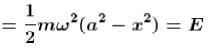Kinetic energy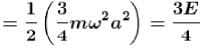*Answer can only contain numeric values
Simple Harmonic Motion NAT Level - 1 - Question 4

The amplitude of a damped harmonic oscillator become halved in 1 minute. After three minutes the amplitude will become 1/x of initial amplitude where x is :

Detailed Solution for Simple Harmonic Motion NAT Level - 1 - Question 4

The variation in amplitude of a damped harmonic oscillation with time is given by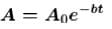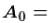initial amplitude,damped factor
It is given that after 1 minutes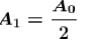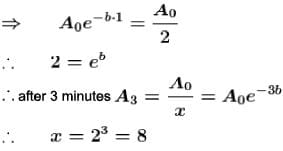*Answer can only contain numeric values
Simple Harmonic Motion NAT Level - 1 - Question 5

One body of mass m is suspended from three springs as shown in figure each spring has spring constant k. If mass m is displaced slightly then time period of oscillation is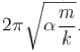Find the value of  α.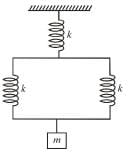Detailed Solution for Simple Harmonic Motion NAT Level - 1 - Question 5

Using series and parallel combination of spring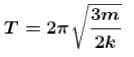*Answer can only contain numeric values
Simple Harmonic Motion NAT Level - 1 - Question 6

A particle is executing SHM with an amplitude 4 cm. The displacement (in cm) at which its energy it half kinetic and half potential is :

Detailed Solution for Simple Harmonic Motion NAT Level - 1 - Question 6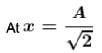Potential energy = Kinetic energy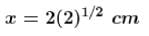*Answer can only contain numeric values
Simple Harmonic Motion NAT Level - 1 - Question 7

A particle executing S.H.M of amplitude 4 cm and T = 4 sec. The time taken (in sec) by it to move from positive extreme position to half the amplitude is :

Detailed Solution for Simple Harmonic Motion NAT Level - 1 - Question 7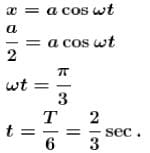*Answer can only contain numeric values
Simple Harmonic Motion NAT Level - 1 - Question 8

The potential energy of a particle executing S.H.M. is 2.5 J when its displacement is half of amplitude. The total energy (in Joule) of the particle is :

Detailed Solution for Simple Harmonic Motion NAT Level - 1 - Question 8

Total energy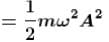Potential energy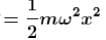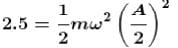So total energy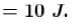*Answer can only contain numeric values
Simple Harmonic Motion NAT Level - 1 - Question 9

If the time period of oscillation of mass M suspended from a spring is one second, then the time period (in sec) of 4M will be :

Detailed Solution for Simple Harmonic Motion NAT Level - 1 - Question 9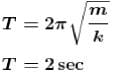*Answer can only contain numeric values
Simple Harmonic Motion NAT Level - 1 - Question 10

A linear harmonic oscillation of force constant 2 x 106 Nlm and amplitude 0.01 m has a total mechanical energy of 160 joules. Its maximum K.E. (in Joule) will be.

Detailed Solution for Simple Harmonic Motion NAT Level - 1 - Question 10

Maximum kinetic energy is 100 J
Maximum kinetic energy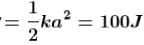## Topic wise Tests for IIT JAM Physics

217 tests
 Use Code STAYHOME200 and get INR 200 additional OFF Use Coupon Code
Information about Simple Harmonic Motion NAT Level - 1 Page
In this test you can find the Exam questions for Simple Harmonic Motion NAT Level - 1 solved & explained in the simplest way possible. Besides giving Questions and answers for Simple Harmonic Motion NAT Level - 1, EduRev gives you an ample number of Online tests for practice

217 tests